# 9 Laws of Technology That Changed the World

Every year, we see various new products and most of them are developed using old technologies. Some influential scientists and mathematicians were born to make a dent in the world. And they successfully did it with their theories, which laid the foundation of modern technology.

The world has changed a lot in the last couple of decades and things that made this possible are based on old theories and inventions. Today, we are presenting you the laws of technology — along with their impact and applications — that played a significant role in changing the world.

### 9. Pythagorean Theorem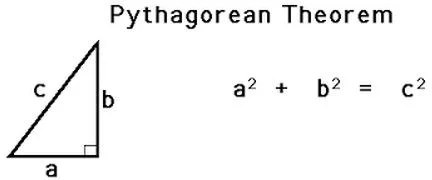History: The Pythagorean theorem was first formulated and solved by a Greek mathematician, Pythagoras. This theorem has now hundreds of proofs. Still, there is no evidence whether the Pythagorean theorem was discovered at once or multiple times by different scientists at different places.

Meaning: It states that the square of the hypotenuse of a right-angle triangle is equal to the sum of squares of the other two sides.

Application: The Pythagorean theorem is regarded as the base of the trigonometry. It also has a major application in the GPS navigation system to accurately survey and create maps.

### 8. Fundamental Theorem of CalculusHistory: The fundamental theorem of calculus is based on differentiation and integration. The first proof of the fundamental theorem was published by James Gregory and Isaac Barry. The present version of the calculus was given by Sir Isaac Newton and Gottfried Leibniz.

Meaning: It is a theorem that interlinks the concept of differentiation and integration. It also allows any calculations that have an instantaneous rate of change.

Application: This theorem has a wide practical application in the field of medicine, economics and computer science. It is used in every equation where an optimal solution is required.

### 7. Logistic Model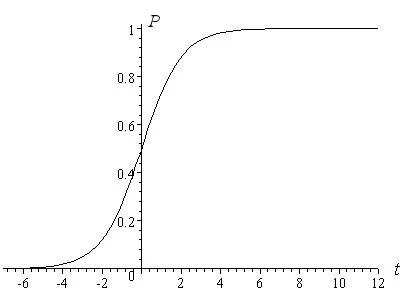History: The logistic function curve was given by Pierre Francois Verhulst in 1844. In the initial stage, the graph is almost exponential and after saturation, growth stops.

Meaning: It is also known as S-shaped curve whose value lies between positive and negative infinity. In its equation, e represents the natural logarithmic number.

Application: It has a wide area of applications in biology, ecology, artificial neural network, probability, statistics, and biomathematics. The model is also used in weather forecasting.

### 6. Shannon Theorem of InformationHistory: The Shannon’s Information Theorem was published in 1948 by Claude Shannon, an engineer at the Bell Lab. It completely changed the way of communication over long distances.

Meaning: Shannon’s Information Theorem estimates the maximum rate at which information can be transferred in the presence of noise. It is a sub-branch of applied mathematics which involves the signal processing operations.

Application: Now this theorem is widely used in lossy and lossless data compression, bioinformatics, music editing, and information retrieval. It has a major impact on the success and development of the internet, CD, mobile phones, and numerous space exploration missions.

### 5. Maxwell’s TheoremsHistory: Michael Faraday, Carl F. Gauss, and Marie Ampere gave three different laws about the connection between electricity and magnetism. James Clerk Maxwell translated these laws into expressions and interrelated them.

Meaning: All four equations of Maxwell show the relationship between electric and magnetic field.

Application: These equations have a large impact on understanding the nature of electromagnetic waves and other technologies. They are still used to make television, radar, and modern communication systems.

### 4. The Fourier Transform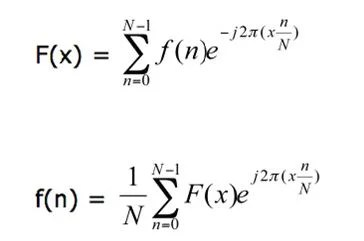History: The Fourier transform was named after his discoverer, Joseph Fourier. He extended the equation of Fourier transform from heat flow and wave equation.

Meaning: It is commonly used to transform a time-based signal to a frequency-based signal. This law defines the signal patterns in time as a function of frequency.

Application: It has several applications in physics, engineering, quantum mechanics and digital signal processing.

### 3. Einstein’s Theory of RelativityHistory: The theory of relativity was discovered by Albert Einstein. It is divided into general and special relativity theory. He used many theoretical results obtained by Hendrik Lorentz, Henri Poincare, and Albert Michelson.

Meaning: It states that the energy released by any object is equal to the product of the mass of the object and square of the speed of light.

Application: It is considered as the most famous equation ever in history. It is used in many electronics systems; a famous example would be the Global Positioning System (GPS).

### 2. Universal Law of Gravitation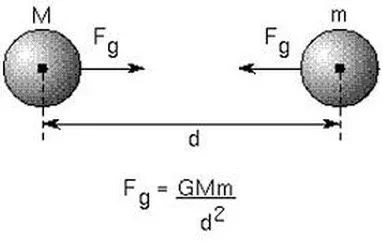History: He used several sources from Johannes Kepler to discover the universal law of gravitation. There are many controversies related to his work including plagiarism of Robert Hooke’s work.

Meaning: According to the universal law of gravitation, the force acting between two bodies in the universe is directly proportional to the product of their masses and inversely proportional to the square of the distance between the bodies. It calculates the force acting between any two objects in the universe.

Application: This equation is used to defy the power of gravity. It is used in space missions to find the minimum force exerted by different bodies. It is also used to find the distance at which satellites are placed.

### 1. Moore’s Law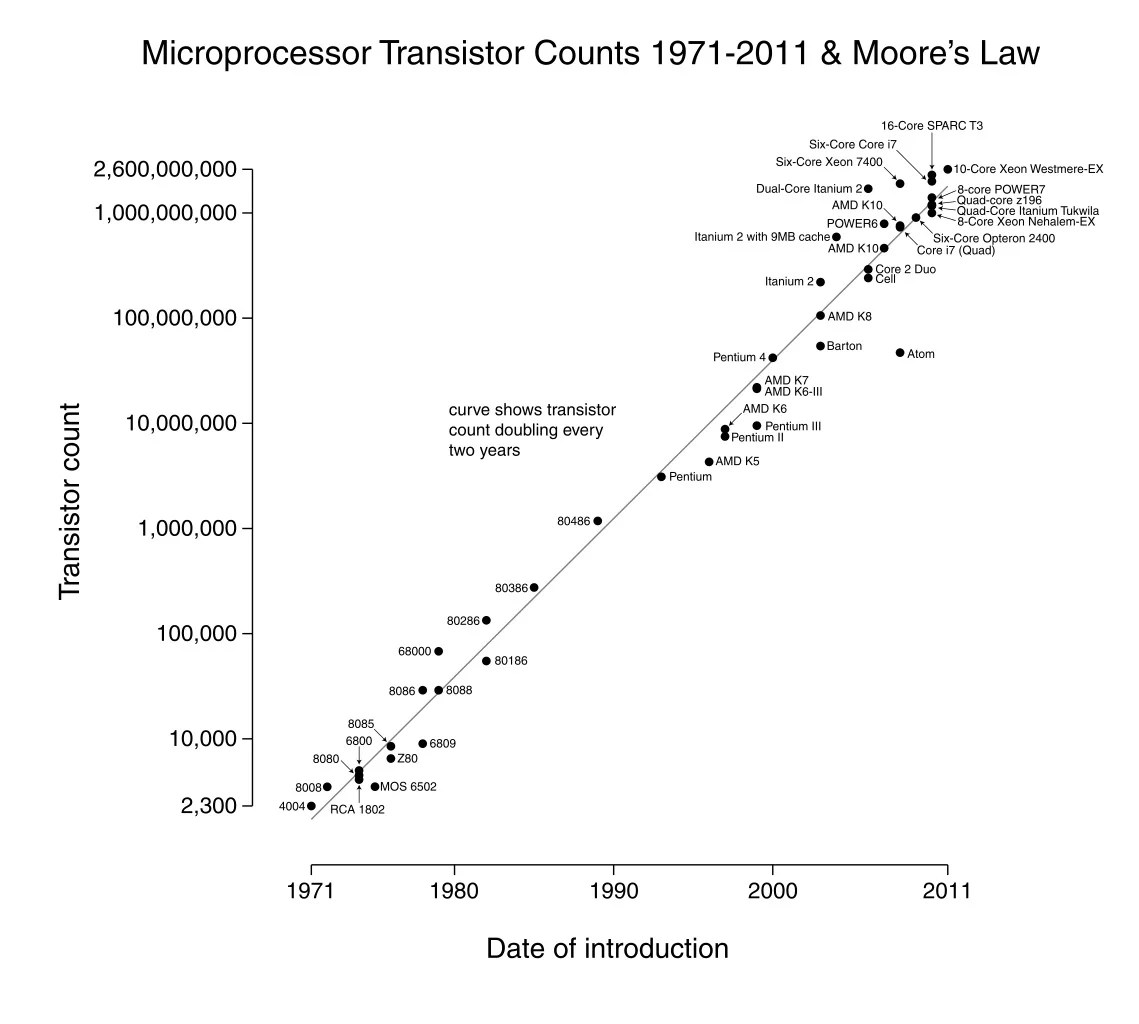History: This law is named after the co-founder of the Intel Corporation, Gordon E. Moore. He makes a prediction in a magazine article in 1965 and now people consider it a law.

Meaning: Gordon E. Moore predicted that the number of transistors in an integrated circuit board doubles every eighteen months. After more than half a century, the law slowed down in 2013 and it is expected that it won’t be true anymore after 2020. It has no equation and usually represented by a linear graph (between a year and a total number of transistors).

Application: This is an observation law, not a natural law. It helped many corporations to set goals for research and development. Somehow, this law also enhanced the market of electronic gadgets all over the world.Written by
###### Varun Kumar

Varun Kumar is a professional science and technology journalist and a big fan of AI, machines, and space exploration. He received a Master's degree in computer science from GGSIPU University. To find out about his latest projects, feel free to directly email him at [email protected]